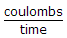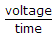# Electronics - Voltage and Current

### Exercise :: Voltage and Current - General Questions

11.

A voltmeter is used:

 A. to measure current B. in series with the circuit C. in parallel with the circuit D. to measure coulombs

Explanation:

No answer description available for this question. Let us discuss.

12.

If the current in a circuit equals 0 A, it is likely that the

 A. voltage is too high B. resistance is too low C. circuit has a short D. circuit is open

Explanation:

No answer description available for this question. Let us discuss.

13.

Current equals:

 A.B. coulombs × time C.D. voltage × time

Explanation:

No answer description available for this question. Let us discuss.

14.

What are the unit and symbol for current?

 A. Ampere, A B. Coulomb, I C. Ampere, Q D. Ampere, I

Explanation:

No answer description available for this question. Let us discuss.

15.

Which part of an atom has no electrical charge?

 A. Electron B. Neutron C. Proton D. All of the above